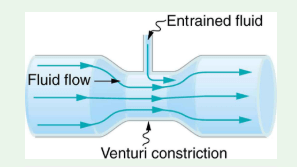MCAT Content / Fluids / Venturi Effect Pitot Tube

### Venturi Effect, Pitot Tube

Topic: Fluids

The Venturi effect is the reduction in fluid pressure when a fluid flows through a constricted section. A pitot tube, a horizontal tube containing a U-shaped tube, is used to determine the velocity of a fluid flowing past it.

pitot tube is a horizontal tube that contains a U-shaped tube. The surface of the pitot tube has at least two openings, one of which faces directly into the fluid flow. The first opening encounters fluid with a velocity of zero. The pressure at this opening is equal to the static pressure exerted by the fluid. The second opening encounters moving fluid. The pressure at this opening is equal to the total pressure exerted by the fluid. Each end of the U-tube is exposed to the pressure at just one of these openings, so the difference in fluid heights withing the U-tube can be used to calculate the differences in pressures according to ΔP=ρgΔh, where ρ is the density of the liquid. Because h1=h2 and v1=0 m/s, Bernoulli’s equation can be simplified to

P2 + ½ρv22 = Por ½ρv22 = P1– P2 = ΔP

If the density of fluid is known, the difference in pressure measured by the U-tube can be used to determine the velocity of the fluid flowing past the pitot tube:Venturi tube is a horizontal tube with a constricted region – a region with decreased cross-sectional area – in its middle. A Venturi tube can be used to determine the velocity of a fluid that is flowing within it. This is in contrast to a pitot tube, which is used to determine the velocity of a fluid flowing past it. The continuity equation states that for a fluid with constant flow rate Q, a decrease in cross-sectional area A is associated with an increase in velocity v. Recall that Av. Bernoulli’s equation states that for a fluid at a constant height, an increase in velocity is associated with a decrease in pressure.

The decrease in pressure occurs when a fluid flows into a constricted region of a pipe is known as the Venturi effect. A venturi meter can be sued to determine the velocity of a fluid in a pipe.Practice Questions

Understanding cardiac pressure-volume curves

The tubular system in an aquarium

MCAT Official Prep (AAMC)

Practice Exam 2 C/P Section Question 25

Key Points

• A pitot tube is a horizontal tube that contains a U-shaped tube. It is used to determine the velocity of a fluid flowing past it.

• A Venturi tube is a horizontal tube with a constricted region – a region with decreased cross-sectional area – in its middle. A Venturi tube can be used to determine the velocity of a fluid that is flowing within it.

• The Venturi effect is the reduction in fluid pressure when a fluid flows through a constricted section.

Key Terms

Pitot tube: a flow measurement device used to measure fluid flow velocity based on Venturi effect. It is widely used to determine the airspeed of an aircraft, water speed of a boat, and to measure liquid, air and gas flow velocities in certain industrial applications.

Venturi tube: A horizontal tube with a constricted region – a region with decreased cross-sectional area – in its middle

Fluid density: The average density of a substance or object is defined as its mass per unit volume, ρ = m/ V.

Bernoulli’s equation: States that for a fluid at a constant height, an increase in velocity is associated with a decrease in pressure.

Venturi effect: The reduction in fluid pressure when a fluid flows through a constricted section.

Billing Information